# Catch up on the latest AI articles# FiLM Solves The Trade-off Between Noise Removal And Variability Detection In Time Series Forecasting Models.

3 main points
✔️ This is a NeurIPS 2022 accepted paper. Separation of noise and signal, a frequent issue in time series forecasting, is effectively exploited by the features of deep learning methods
✔️ Specifically, Legendre polynomial projection is applied to approximate historical information, Fourier projection is used to remove noise, and low-rank approximation is added to speed up the computationWe propose FiLM
✔️ which significantly improves the accuracy of the state-of-the-art model (20.3% and 22.6%) for multivariate and univariate long-term forecasts, respectively. It is also worth mentioning that it can be used as a plugin to other deep learning modules

FiLM: Frequency improved Legendre Memory Model for Long-term Time Series Forecasting
written by
(Submitted on 18 May 2022 (v1), last revised 16 Sep 2022 (this version, v4))
Comments: Accepted by The Thirty-Sixth Annual Conference on Neural Information Processing Systems (NeurIPS 2022)
Subjects:
Machine Learning (cs.LG); Machine Learning (stat.ML)code：The images used in this article are from the paper, the introductory slides, or were created based on them.

## summary

Recent studies have shown that deep learning models such as RNNs and Transformers can provide significant performance gains for long-term prediction of time series by making effective use of historical information. However, we found that there is still significant room for improvement in how to store historical information in neural networks while avoiding overfitting to the noise that appears in the history. Solving this problem will allow us to better utilize the capabilities of deep learning models.

For this purpose, this paper designs a frequency improved Legendre memory model (FiLM: Frequency improved Legendre Memory). It applies a Legendre polynomial projection to approximate historical information, uses a Fourier projection to remove noise, and adds a low-rank approximation to speed up the computation.

Empirical studies show that the proposed FiLM significantly improves the accuracy of state-of-the-art models (20.3% and 22.6%) in multivariate and univariate long-term prediction, respectively. We also demonstrate that the representation module developed in this study can be used as a general plug-in to improve the long-term prediction performance of other deep learning modules.

## Introduction.

Long-term forecasting differs from short-term forecasting in that it is based on a long range of future history. Long-term time series forecasting has many important applications, including energy, weather, economics, and transportation. It is more challenging than regular time series forecasting. Challenges include long-term time dependence, ease of error propagation, complex patterns, and nonlinear dynamics. These challenges make accurate forecasting generally impossible with traditional learning methods such as ARIMA.

Deep learning methods such as RNNs have made breakthroughs in time series prediction, but Rangapuram et al. (2018); Salinas et al. (2020), Pascanu et al. (2013) often suffer from problems such as gradient loss/explosion, which limits their practical performance NLP and Following the success of Transformer Vaswani et al. (2017) in the CV community, Wen et al. (2022b), Zhou et al. (2022), Wu et al. (2021), and Zhou et al. (2021) show promising performance in capturing long-term dependence for time series prediction.

To achieve accurate predictions, many deep learning researchers have increased the complexity of their models in hopes of capturing important and complex historical information. However, these methods have not been able to achieve their goals.Fig. 1 shows a grand-truth time series of the real-world ETTm1 dataset and the vanilla transformer model Vaswani et al. (2017) and LSTM models Hochreiter & Schmidhuber (1997a) predictions are compared. We see that the predictions deviate completely from the grand-truth distribution. We believe that these errors stem from the fact that these models mis-capture noise while trying to preserve the true signal.

The authors conclude that there are two keys in accurate forecasting:

1) How to capture important historical information as completely as possible

(2) How to effectively remove noise

Therefore, in order to avoid derailing the forecast, we could not simply improve the model by making it more complex; instead, we decided to consider a robust representation that could capture the important patterns in the Wen et al. (2022a) time series without noise.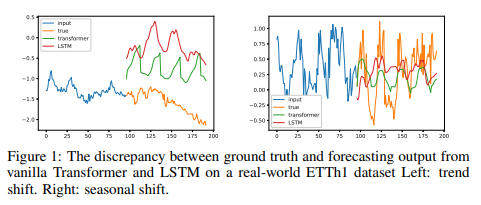This observation motivated us to switch our perspective from long time series projections to long sequence compression. Recursive memory models have achieved impressive results in the function approximation task: recursive memory units (LMUs) with Legendre projection provide a good representation for long time series; the S4 model has come up with another recursive memory design for data representation and has significantly improved the state-of-the-art results of the long range prediction benchmark (LRA ) state-of-the-art results for long-range forecasting benchmarks (LRA), which is a significant improvement. However, when it comes to long-range time series forecasting, these approaches have not been able to match the state-of-the-art performance of Transformer-based methods.

Careful examination reveals that these data compression methods are more powerful in recovering details of historical data than the LSTM/Transformer model, as revealed in Fig. 2. However, their tendency to overfit all past spikes makes them vulnerable to noisy signals and limits their long-term predictive performance; it is worth noting that the Legendre Polynomials employed in LMP are only a special case within the orthogonal polynomial (OPs) family OPs (including Legendre, Laguerre, Chebyshev, etc.) and other orthogonal bases (Fourier and Multiwavelets) have been extensively studied in numerous fields and recently applied in deep learning.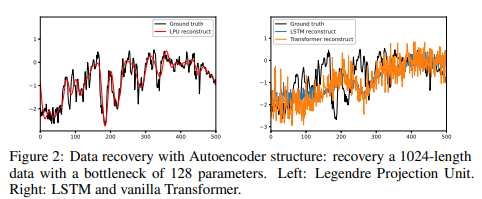These observations indicate the need to develop accurate and robust methods for representing time-series data for future forecasting, especially for long-term forecasting. The proposed method integrates those representations with a powerful forecasting model and significantly outperforms existing long-term forecasting methods on multiple benchmark data sets.

As a first step toward this goal, we directly exploit the Legendre projection used by LMU Voelker et al. (2019) to dynamically update the representation of time series with fixed-size vectors. We then combine this projection layer with various deep learning modules to improve prediction performance. The main challenge in directly utilizing this representation is the dilemma between preserving information and overfitting data, i.e., the larger the number of Legendre projections, the more historical data is preserved, but noisy data is more likely to be overfitted.

Therefore, as a second step, we introduce a layer of dimensionality reduction that combines Fourier analysis and low-rank matrix approximation to reduce the impact of noisy signals on the Legendre projection. Specifically, a large dimensional representation is left out of the Legendre projection so that all important details of the historical data are preserved. We then apply a combination of Fourier analysis and low-rank approximation to preserve the portion of the representation associated with the low-frequency Fourier components and the upper eigenspace to remove the effects of noise.

Thus, it not only captures long-term time dependence, but also effectively reduces noise in long-term forecasts. The authors named the proposed method Frequency improved Legendre Memory model for long-term time series forecasting, or FiLM for short.

The major contributions of this study can be summarized as follows

1. propose a mixed-expert frequency-improved Legendre memory model (FiLM) architecture for robust multi-scale time series feature extraction

2. redesign the Legend Projection Unit (LPU) to make it a general tool for data representation that can be used by time series forecasting models to solve historical information storage problems

3. propose a frequency extension layer (FEL) that combines Fourier analysis and low-rank matrix approximation to reduce dimensionality, minimize the effect of noise signals from time series, and mitigate overfitting problems

4. conduct large-scale experiments on six benchmark datasets across multiple domains (energy, transportation, economy, weather, disease).

Empirical studies show that the proposed model improves the performance of state-of-the-art methods by 19.2% and 26.1% in multivariate and univariate forecasting, respectively. The empirical study also revealed a dramatic improvement in computational efficiency due to dimensionality reduction.

## Time series representation in the Legendre-Fourier domain

### Legendre Projection

Legend polynomial projection allows for the projection of long time data sequences onto a bounded dimensional subspace, leading to a compressed or feature representation of the evolving historical data. Formally, given a smooth function f observed online, the goal is to maintain a fixed-size compressed representation of the history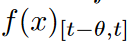, where θ specifies the window size. At each time point t, an approximate function g (t)( x) is defined with respect to the measure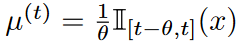. In this paper, Legend polynomials of order at most N 1 are used to construct the function g (t) (x).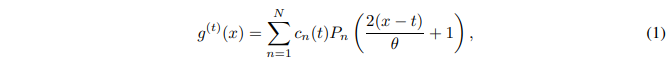where Pn(⋅) is the nth-order Legendre polynomial. The coefficients cn ( t) are captured by the following dynamic equation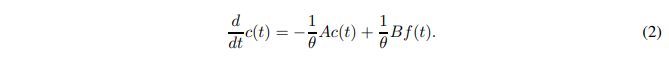where the definitions of A and B can be found in Voelker et al. (2019). Using Legendre polynomials as a basis allows for an exact approximation of smooth functions, as shown in the following theorem.

Theorem 1

If f (x) is L-Lipschitz,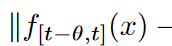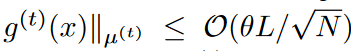It follows that f (x) is a bounded derivative of order k. Furthermore, if f (x) has kth-order bounded derivatives,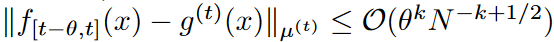The first is the "A" in the "A" column.

According to Theorem 1, naturally, the higher the number of bases of the Legendre polynomial, the higher the approximation accuracy, which unfortunately may lead to overfitting of the noise signal in the history. As mentioned above, feeding the above features as they are to deep learning modules such as MLP, RNN, and vanilla attention, etc., will not provide state-of-the-art performance, mainly due to the noise signal in the history. Therefore, we next introduce a Frequency Enhanced Layer with Fourier Transform for feature selection.

Theorem 2

Let A be a unitary matrix and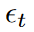be 2-sub Gaussian random noise.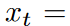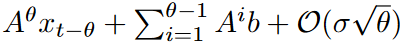The following is a summary of the results of the study.

### Fourier transform

Since white noise has a perfectly flat power spectrum, it is commonly believed that time series data enjoy a particular spectral bias and are not generally randomly distributed across the spectrum. Because of the stochastic transition environment, the actual output trajectories of the forecasting task contain a great deal of volatility, and people usually only predict their average path. Therefore, relatively smooth solutions are preferred.

According to equation (1), the approximate function g (t) (x) can be stabilized by smoothing the coefficients cn ( t) by both t and n. This observation helps to design an efficient data-driven method for adjusting the coefficients cn(t), since smoothing over n can be easily implemented by multiplying each channel by a scalar that can be learned, and here we mainly discuss smoothing cn ( t) over t by the Fourier transform.

The spectral bias means that the spectrum of cn(t) is mainly located in the low-frequency region and the signal intensity is weak in the high-frequency region. To simplify the analysis, we will assume that the Fourier coefficient of cn(t) is an(t). For spectral bias, we assume that there exists s, amin > 0 such that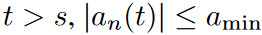is satisfied for all n. An idea for sampling the coefficients is to keep the first k dimensions and randomly sample the remaining dimensions instead of a completely random sampling strategy. The authors characterize the approximation quality by the following theorem

Theorem 3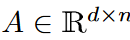Let Ω(k/n) be the Fourier coefficient matrix of the input matrix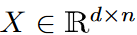and µ(A) the coherence measure of matrix A. Assume that there exist s and positive amin such that the elements of the last d-s column of A are smaller than amin. Keeping the first s column selected and randomly selecting O( k2/ε2 -s) columns from the rest, we obtain with high probability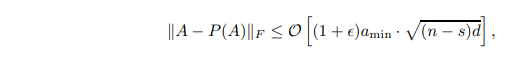where P(A) denotes the matrix projecting A onto the column-selected column space.

Theorem 3 implies that when amin is sufficiently small, the selected space can be regarded as almost identical to the original space.

## Model Structure

### FiLM: Frequency improved Legendre Memory Model

The overall structure of the FiLM is shown in Fig. 3. FiLM maps the sequence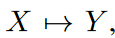by utilizing two main sublayers. Here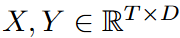. That is, it mainly uses two sublayers: the LPU (Legendre Projection Unit) layer and the FEL (Fourier Enhanced Layer) layer. In order to capture historical information at different scales, the LPU layer is implemented with a mix of different scale specialists. An optional add-on data normalization layer, RevIN Kim et al. (2021), has been introduced to further enhance the robustness of the model; FiLM is a simple model with only one LPU and one FEL layer each.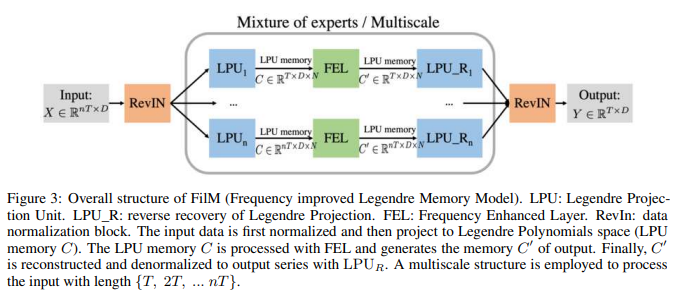### LPU: Legendre Projection Unit

LPU is a state-space model: Ct = ACt-1 + Bxt , where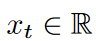is the input signal,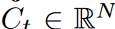is the memory unit, and N is the number of Legend polynomials. LPU contains two untrainable prematrices A and B defined as follows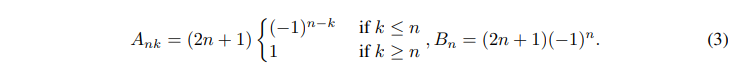LPU has two stages: projection and reconstruction. The former stage projects the original signal to the memory unit: C = LPU(X). The latter stage reconstructs the signal from the memory unit: Xre = LPU_R(C). The entire process of projecting/reconstructing the input signal to/from memory C is shown in Fig. 4.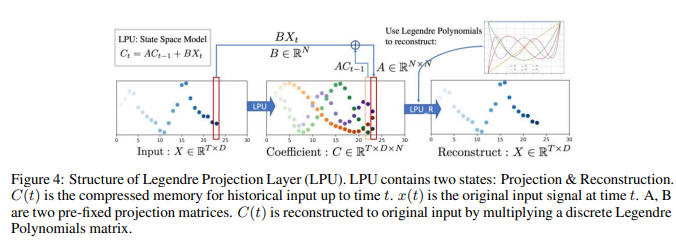### FEL: Frequency Enhanced Layer

Low Approximation

FEL has a single trainable weights matrix (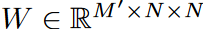) that is needed to learn from the data. However, this weight can be large; we can decompose W into three matrices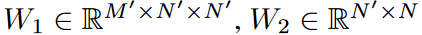,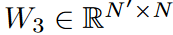for a low-rank approximation (N '<< N ). Using the Legend polynomial number N = 256 as the default, the trainable weights for this model are significantly reduced to 0.4% at N' = 4, with little degradation in accuracy. The calculation scheme is shown in Fig. 5.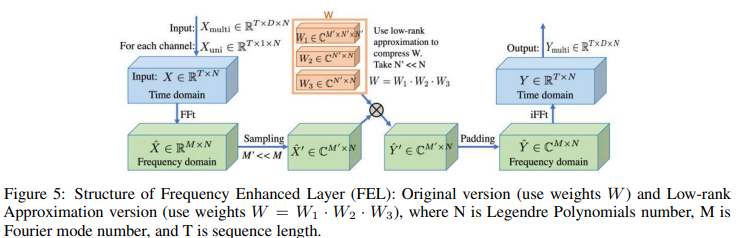Mode Selection

To reduce noise and increase training speed, select a subset of frequency modes after the Fourier transform. The default selection policy is to select the lowest M mode. In our experiments, we considered various selection policies. The results show that for some data sets, adding random high-frequency modes can provide additional improvement (supported by theoretical studies in Theorem 3).

### Mixing Mechanisms for Multiscale Experts

Multiscale phenomena are an important data bias inherent in time series forecasting. The model in this paper may lack such a prior distribution, since the authors uniformly emphasize points in the historical sequence. As shown in Fig. 3, this model is based on the use of input sequences with various time horizons {T, 2T, . nT}, we implemented a simple mixed-expert strategy that utilizes input sequences with time horizon T to predict the prediction horizon T and merges each expert prediction in a linear layer. This mechanism consistently improved the performance of the model across all datasets, as shown in Table 7.

### data normalization

As Wu et al. (2021); Zhou et al. (2022) point out, seasonal trend decomposition of time series is an important data normalization design in long-term time series forecasting. The authors find that this LMU projection can essentially play the role of normalization for most data sets, but the lack of an explicit normalization design may compromise the robustness of the performance in some cases. Simple reversible instance normalization (RevIN) Kim et al. (2021) is adapted to serve as an add-on explanatory data normalization block. Means and standard deviations are computed for all instances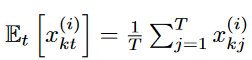and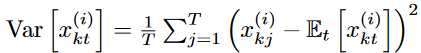. x(i) as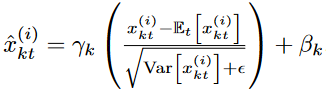for all instances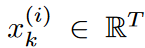of the input data.

The normalized input data is then sent to the model for prediction. Finally, the model output is denormalized by applying the inverse of the initial normalization.

RevIN slows the learning process by a factor of 2 to 5, but it also does not mean that consistent improvements are observed in all data sets when RevIn is applied. Therefore, RevIn can be considered an optional stabilizer in model learning. Its detailed performance is shown in the truncation study in Table 7.

## experiment

To evaluate FiLM, the authors conducted extensive experiments on six real-world benchmark datasets popular for long-term forecasting, including transportation, energy, economics, weather, and disease. The authors primarily used five state-of-the-art (SOTA) Transformer-based models: the FEDformer, Autoformer Wu et al. (2021), Informer Zhou et al. (2021), LogTrans Li et al. (2019), Reformer Kitaev et al. 2020), and a recent state-space model with recursive memory, S4 Gu et al. (2021a), for comparison; FEDformer is chosen as the main baseline because it achieves SOTA results in most settings.

### Main Results

For better comparison, we followed the experimental setup of Informer Zhou et al. (2021), adjusting the input length for best prediction performance and fixing the prediction lengths for both training and evaluation to 96, 192, 336, and 720, respectively.

Multivariate

For the multivariate forecasting task, FiLM achieved the best performance across the full range for all six benchmark datasets, as shown in Table 1. compared to the SOTA work (FEDformer), the proposed FiLM was able to yield an overall relative MSE reduction of 20.3%. overall relative MSE reduction of 20.3%. Notably, for some datasets, such as Exchange, an even greater improvement was observed (>30%). axes consistently, demonstrating the strength of the FiLM in long-term forecasting.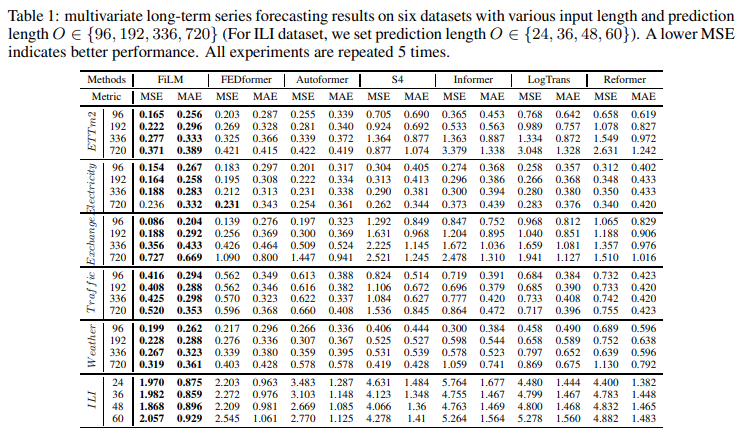Univariate

Benchmark results for univariate time series forecasts are summarized in Table 10: Compared to the SOTA work (FEDformer), FiLM achieved an overall relative MSE reduction of 22.6%. It was also able to reach improvements of more than 40% on some data sets, such as weather and electricity demand. This once again demonstrates the effectiveness of FiLM in long-term forecasting.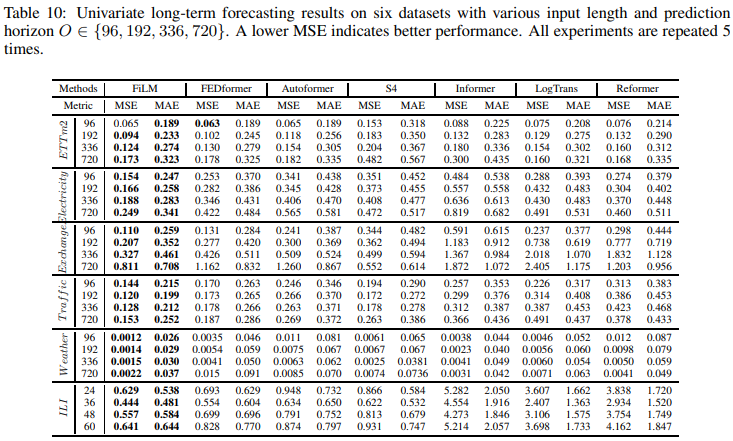LPU boosting results

As shown in Fig. 6, a series of experiments were conducted to measure the boosting effect of combining LPU with various popular deep learning modules (MLP, LSTM, CNN, Attention). The experiments compared LPUs with linear layers of the same size; it is worth noting that LPUs do not contain any trainable parameters. Results are shown in Table 19. For all modules, LPU significantly improved the average performance in long-term prediction: mlp: 119.4%, lstm: 97.0%, cnn: 13.8%, Attention: 8.2%. vanilla Attention, when combined with LPU, showed relatively performance and is worth further exploration.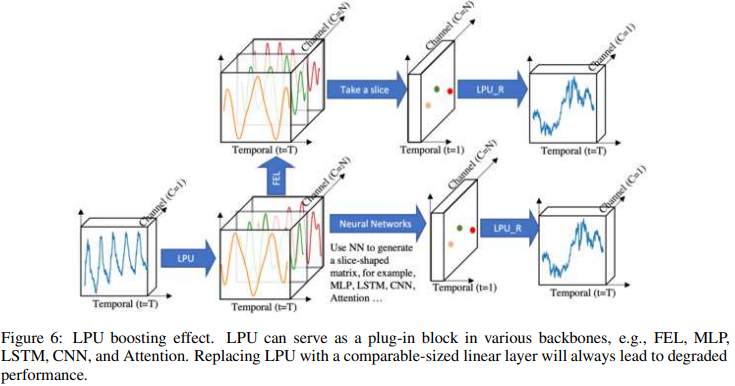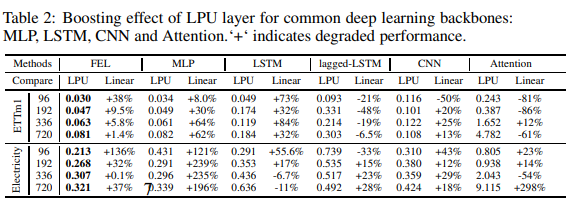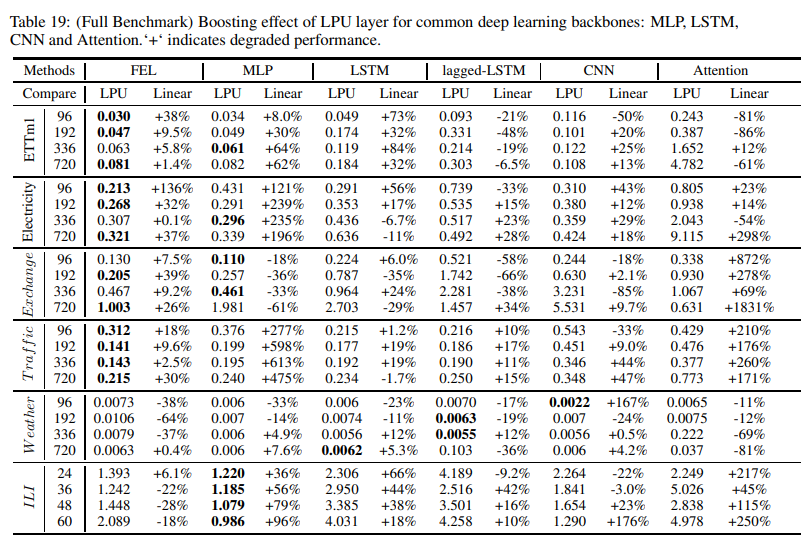Lowest approximation of FEL

A low-rank approximation of the trainable matrix in the frequency extension layer can significantly reduce the parameter size to 0.1%⇠0.4% with a slight accuracy degradation. Details of the experiments are shown in Table 3; compared to the Transformer-based baseline, FiLM can reduce the number of learnable parameters by 80% and memory usage by 50%.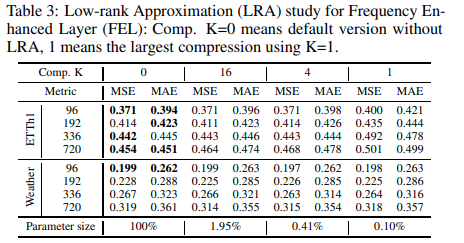FEL's Mode Selection Policy

The frequency mode selection policy is considered in Table 4; the Lowest mode selection method shows the most robust performance; the results in the Low random column show that, as supported by the theoretical work in Theorem 3, adding some high-frequency signals at random yields special improvements on some data sets The results in the Low random column show that adding some high-frequency signals at random yields special improvements on some data sets, as supported by the theoretical work in Theorem 3.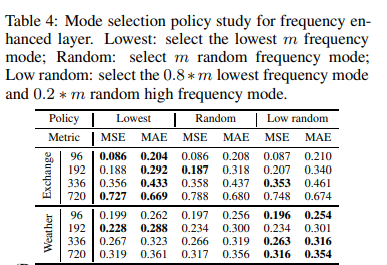### sectional analysis

This section presents the two main blocks employed (FEL & LPU) isolation studies, the multi-scale mechanism, and data normalization (RevIN).

Isolation of LPUs

To prove the effectiveness of the LPU layer, Table 5 compares the original LPU layer with six variations: the LPU layer consists of two sets of matrices (projection matrix and reconstruction matrix). Each has three variations: fixed, trainable, and random-initial.

Variation 6 uses a linear layer of equivalent size to replace the LPU layer. Variation 6 resulted in an average degradation of 32.5%, confirming the effectiveness of the Legendre projection: the LPU projection matrix is called recursively N times (where N is the length of the input). Therefore, if the projection matrix is initialized randomly (Variation 5), the output will face the problem of exponential explosion. If the projection matrix is learnable (variants 2, 3, and 4), the model suffers from the same exponential explosion and must be trained at a small learning rate, which is slow and takes many epochs to converge. Thus, given the tradeoff between speed and performance, the learnable projection version is not recommended. The variant with a learnable reconstruction matrix (variant 1) has comparable performance and is less difficult to converge.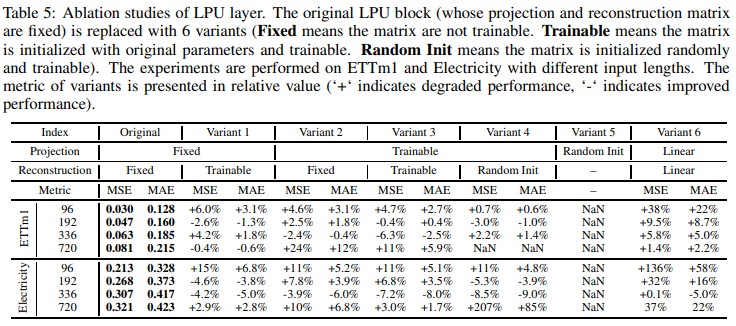Isolation of FEL

To prove the effectiveness of FEL, we replaced it with several variants (MLP, LSTM, CNN, Transformer). S4 was also introduced as a variant because it has a version with Legendre projected memory. Experimental results are summarized in Table 6: FEL achieves the best performance compared to LSTN, CNN, and Attention; MLP achieves comparable performance for input lengths of 192, 336, and 720. However, MLP has a memory usage of N 2L (FEL is N 2), which is not tolerable; S4 achieved similar results to LPU+MLP. LPU+CNN also performed the worst.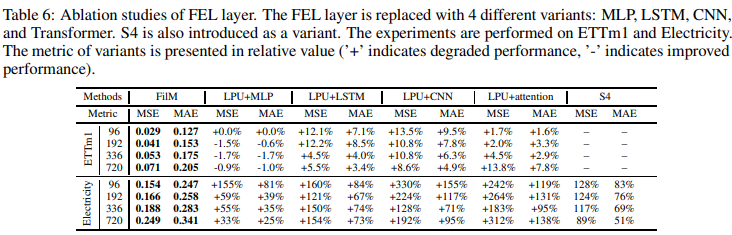Separation ofmulti-scale and data normalization (RevIN)

The multiscale module consistently yields significant improvements across all data sets. However, we found mixed performance, with data normalization improving performance for "Traffic" and "Disease," but only marginal improvements for the rest Table 7 shows an ablation study employing a mixture of RevIN data normalization and multiscale expert .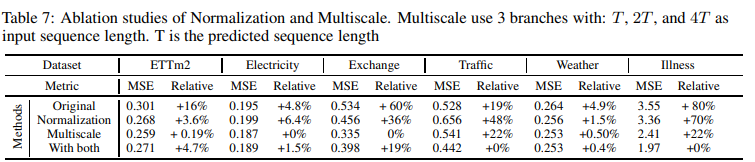## summary

In long-term forecasting, the trade-off between preserving historical information and removing noise is an important issue for accurate and robust forecasting. To address this challenge, the authors proposed the Frequency Improved Legendre Memory Model (FiLM), which accurately preserves historical information and removes noise signals. Furthermore, the authors theoretically and empirically proved the effectiveness of the Legendre and Fourier projections employed in this model.

Extensive experiments have shown that the proposed model significantly improves SOTA accuracy on six benchmark data sets. Of particular importance is that the proposed framework is fairly general and can be used as a building block for long-term projections in future studies. It can also be modified for different scenarios. For example, the Legendre Projection Unit can be replaced by other orthogonal functions such as Fourier, Wavelets, Laguerre Polynomials, Chebyshev Polynomials, etc.

Based on the noise properties, the Fourier Enhanced Layer proved to be one of the best candidates for this framework. The authors plan to consider further variants of this framework in the future.

If you have any suggestions for improvement of the content of the article,